## GATE 2021 CS-Set-2

 Question 1
Listening to music during exercise improves exercise performance and reduces discomfort. Scientists researched whether listening to music while studying can help students learn better and the results were inconclusive. Students who needed external stimulation for studying fared worse while students who did not need any external stimulation benefited from music. Which one of the following statements is the CORRECT inference of the above passage?
 A Listening to music has no effect on learning and a positive effect on physical exercise. B Listening to music has a clear positive effect on physical exercise. Music has a positive effect on learning only in some students. C Listening to music has a clear positive effect both on physical exercise and on learning. D Listening to music has a clear positive effect on learning in all students. Music has a positive effect only in some students who exercise.
Aptitude       Verbal       Video-Explanation
Question 1 Explanation:

From the first statement “Listening to music during exercise improves exercise performance and reduces discomfort. “ It is clear that listening to music has a positive effect on physical exercise.

From the statement “Scientists researched whether listening to music while studying can help students learn better and the results were inconclusive. “   it is clear that only on some students music has a positive effect.

 Question 2A transparent square sheet shown above is folded along the dotted line. The folded sheet will look like _______.
 ABCDAptitude       Non-Verbal       Video-Explanation
Question 2 Explanation:
Fold the sheet along the dotted line and join the shapes, we get option 2.
 Question 3
Gauri said that she can play the keyboard _______ her sister.
 A as worse as B as well as C as better as D as nicest as
Aptitude       Verbal       Video-Explanation
Question 3 Explanation:
‘worse’, ‘better’ are comparative words which need the word ‘than’ for comparison. Out of the remaining two options ‘as well as’ is the correct answer.
 Question 4
Pen : Write :: Knife : _______ Which one of the following options maintains a similar logical relation in the above?
 A Vegetables B Sharp C Blunt D Cut
Aptitude       Verbal       Video-Explanation
Question 4 Explanation:
A pen is used to write. Similarly, a knife is used to cut.
 Question 5
The number of students in three classes is in the ratio 3:13:6. If 18 students are added to each class, the ratio changes to 15:35:21. The total number of students in all the three classes in the beginning was:
 A 66 B 22 C 88 D 110
Aptitude       Numerical       Video-Explanation
Question 5 Explanation:

Given Initial ratio : 3:13:6

Total number of students initially = 3x+13x+6x = 22x

From the given question, we can write 3x+18 = 15y -----I

13x+18 = 35y-----II

6x+18 = 21y------III

I - II => -10X = -20Y

X=2Y------IV

Put IV in III => 6(2y) + 18 = 21y

12y + 18 = 21y

9y = 18

y = 2 -----V

Substitute V in I => 3x + 18 = 30

3x = 12

x = 4

Number of initial students = 22x = 22(4) = 88

 Question 6The number of units of a product sold in three different years and the respective net profits are presented in the figure above. The cost/unit in Year 3 was ₹ 1, which was half the cost/unit in Year 2. The cost/unit in Year 3 was one-third of the cost/unit in Year 1. Taxes were paid on the selling price at 10%, 13% and 15% respectively for the three years. Net profit is calculated as the difference between the selling price and the sum of cost and taxes paid in that year.
The ratio of the selling price in Year 2 to the selling price in Year 3 is ______.
 A 1:1 B 3:4 C 1:2 D 4:3
Aptitude       Numerical       Video-Explanation
Question 6 Explanation:

According to the given data:

Year     Cost/unit   Number of units     Cost Price

1           ₹ 3                    100                     300

2           ₹ 2                     200                    400

3           ₹ 1                     300                    300

Cost Price = Cost/unit x Number of units

Net Profit = Selling Price - (Cost Price + (Tax%  x selling price )

Year 2:

296 = SP - (400 + (13/100) x SP)

=>296 = 0.87SP - 400

=> 0.87SP = 696

=> SP = ₹ 800

Year 3:

210 = SP - (300 + (15/100) x SP)

=> 210 = 0.85SP - 300

=>  0.85SP = 510

=> SP = ₹ 600

The ratio of selling price in Year 2 to the selling price in year 3 = 800:600

= 4:3

 Question 7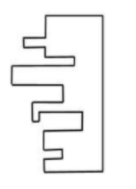A jigsaw puzzle has 2 pieces. One of the pieces is shown above. Which one of the given options for the missing piece when assembled will form a rectangle? The piece can be moved, rotated or flipped to assemble with the above piece.
 ABC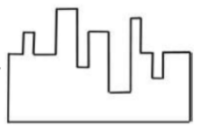DAptitude       Non-Verbal       Video-Explanation
Question 7 Explanation:
Rotate 90 degrees to the left and flip the piece in Option 2.
 Question 8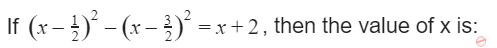A 2 B 4 C 8 D 6
Aptitude       Numerical       Video-Explanation
Question 8 Explanation:

Expand the given equation:

x2+1/4 - x -x2 -9/4 +3x = x+2

=> -2 + 2x = x + 2

X = 4

 Question 9
If θ is the angle, in degrees, between the longest diagonal of the cube and any one of the edges of the cube, then, cos θ =
 AB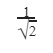CDAptitude       Numerical       Video-Explanation
Question 9 Explanation: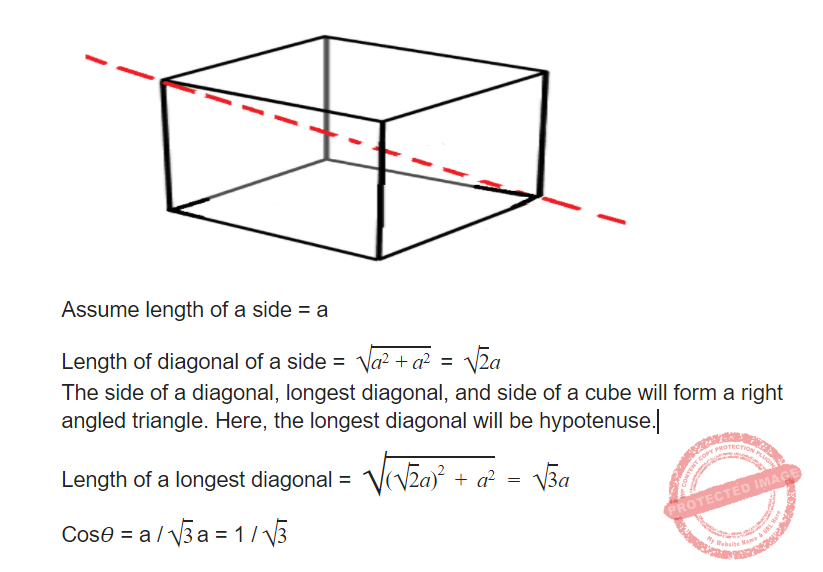Question 10
Six students P, Q, R, S, T and U, with distinct heights, compare their heights and make the following observations.
Observation I: S is taller than R.
Observation II: Q is the shortest of all.
Observation III: U is taller than only one student.
Observation IV: T is taller than S but is not the tallest.
The number of students that are taller than R is the same as the number of students shorter than _______.
 A S B P C T D R
Aptitude       Verbal       Video-Explanation
Question 10 Explanation:
The order will be PTSRUQ
Number of students taller than R = Number of students shorter than S = 3
 Question 11
Consider the three-way handshake mechanism followed during TCP connection established between hosts P and Q. Let X and Y be two random 32-bit starting sequence numbers chosen by P and Q respectively. Suppose P sends a TCP connection request message to Q with a TCP segment having SYN bit = 1, SEQ number = X, and ACK bit = 0. Suppose Q accepts the connection request. Which one of the following choices represents the information present in the TCP segment header that is sent by Q to P?
 A SYN bit = 1, SEQ number = X+1, ACK bit = 0, ACK number = Y, FIN bit = 0 B SYN bit = 0, SEQ number = X+1, ACK bit = 0, ACK number = Y, FIN bit = 1 C SYN bit = 1, SEQ number = Y, ACK bit = 1, ACK number = X+1, FIN bit = 0 D SYN bit = 1, SEQ number = Y, ACK bit = 1, ACK number = X, FIN bit = 0
Computer-Networks       Sliding-Window-Protocol       Video-Explanation
Question 11 Explanation:

Q will send the SYN bit = 1 to the connection establishment.

Q Seq number will be Y different from X

ACK bit = 1 because sending the ACK

ACK number = X+1 (Next seq number id)

FIN bit = 0 (Because establishing the connection)

 Question 12
Which of the following regular expressions represent(s) the set of all binary numbers that are divisible by three? Assume that the string ∊ is divisible by three.
 A (0+1 (01*0)*1)* B (0+11+10(1+00)*01)* C (0*(1(01*0)*1)*)* D (0+11+11(1+00)*00)*
Theory-of-Computation       Regular-Expression       Video-Explanation
Question 12 Explanation:

Transition table

 0 1 ->*q0 q0 q1 q1 q2 q0 q2 q1 q2

DFA of divisible by 3The regular expression will be

Three paths to reach to final state from initial state

Path 1: self loop of 0 on q0

Path 2: q0->q1->q0  hence 11

Path 3: q0->q1->q2->q1->q0 hence 10(1+00)*01

So finally the regular expression will be

(0+11+10(1+00)*01)*

Other regular expression is (if we consider two paths)

Path 1: Path 1: self loop of 0 on q0

Path 2: q0->q1->q2*->q1->q0

Hence regular expression

(0+1 (01*0)*1)*

Another regular expression is (if we consider only one path and remaining part is present inside this path as cycles)

q0->q1->q2->q1->q0

Another regular expression is

(0*(1(01*0)*1)*)*

So A,B, C are correct.

Option D generates string 11100 which is not accepted by FA hence wrong.

 Question 13
For a string w, we define wR to be the reverse of w. For example, if w = 01101 then wR= 10110. Which of the following languages is/are context-free?
 A L={w x wR xR | w, x ∈ {0,1}* } B L={w wR x xR | w, x ∈ {0,1}* } C L={w x xR wR | w, x ∈ {0,1}* } D L={w x wR | w, x ∈ {0,1}* }
Theory-of-Computation       Context-Free-Language       Video-Explanation
Question 13 Explanation:

Option A: L={w x wR  xR  | w, x ∈ {0,1}* }

This is not CFL as if we push “w” then “x” then we cannot match wR with “w” as top of stack contains x.

Option B: L={w wR x xR  | w, x ∈ {0,1}* }

This is CFL. We non deterministically guess the middle of the string. So we push “w” then match with wR  and again push x and match with xR

Option C: L={w x  xR wR   | w, x ∈ {0,1}* }

This is also CFL. We non deterministically guess the middle of the string. So we push “w” then push x and then  match with xR  and again match with wR

Option D: L={w x  wR   | w, x ∈ {0,1}* }

This is a regular language (hence CFL). In this language every string start and end with same symbol (as x can expand).

 Question 14
For a statement S in a program, in the context of liveness analysis, the following sets are defined:
USE(S) : the set of variables used in S
IN(S)     : the set of variables that are live at the entry of S
OUT(S)  : the set of variables that are live at the exit of S
Consider a basic block that consists of two statements, S1followed by S2. Which one of the following statements is correct?
 A OUT(S1) =IN(S2) U OUT(S2) B OUT(S1) =IN(S1) U USE(S1) C OUT(S1) =USE(S1) U IN(S2) D OUT(S1) =IN(S2)
Compiler-Design       liveness-analysis       Video-Explanation
Question 14 Explanation:
As we know the formula for In and OUT are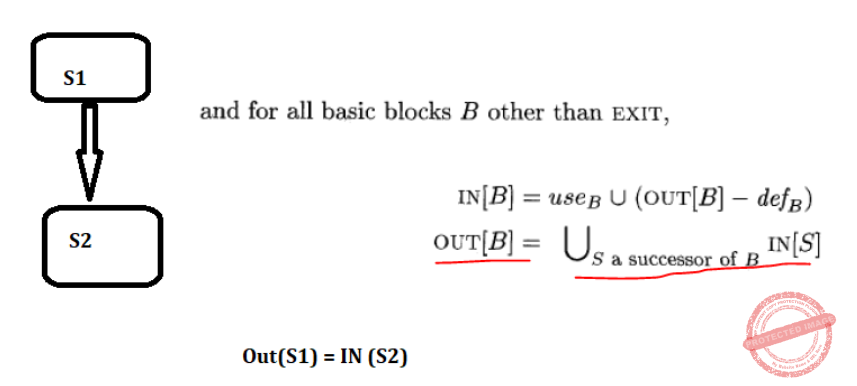Question 15
A data consisting of 1,50,000 student-records is stored on a hard disk with block size of 4096 bytes. The data file is sorted on the primary key RollNo. The size of a record pointer for this disk is 7 bytes. Each student-record has a candidate key attribute called ANum of size 12 bytes. Suppose an index file with records consisting of two fields, ANum value and the record pointer to the corresponding student record, is built and stored on the same disk. Assume that the records of data file and index file are not split across disk blocks. The number of blocks in the index file is ______.
 A 698
Database-Management-System       Indexing       Video-Explanation
Question 15 Explanation:

Total no. of records = 150000

Block size = 4096 bytes

Key size = 12 bytes

Record pointer size = 7 bytes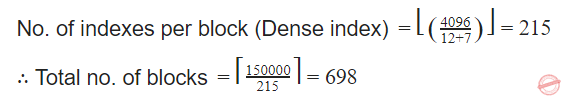Question 16
Consider the following ANSI C program.
#include <stdio.h>
int foo(intx, int y, int q)
{
if ((x <= 0) && (y <= 0))
return q;
if (x <= 0)
return foo(x, y-q, q);
if (y <= 0)
return foo(x-q, y, q);
return foo(x, y-q, q) + foo(x-q, y, q);
}
int main()
{
int r = foo(15, 15, 10);
printf(“%d”, r);
return 0;
}
The output of the program upon execution is ______
 A 60
Programming       Recursion       Video-Explanation
Question 16 Explanation:

int foo(intx, int y, int q)

{

if ((x <= 0) && (y <= 0))  //if 1

return q;

if (x <= 0)                      //if 2

return foo(x, y-q, q);

if (y <= 0)                     //if 3

return foo(x-q, y, q);

return foo(x, y-q, q) + foo(x-q, y, q);

}

int main()

{

int r = foo(15, 15, 10);

printf(“%d”, r);

return 0;

}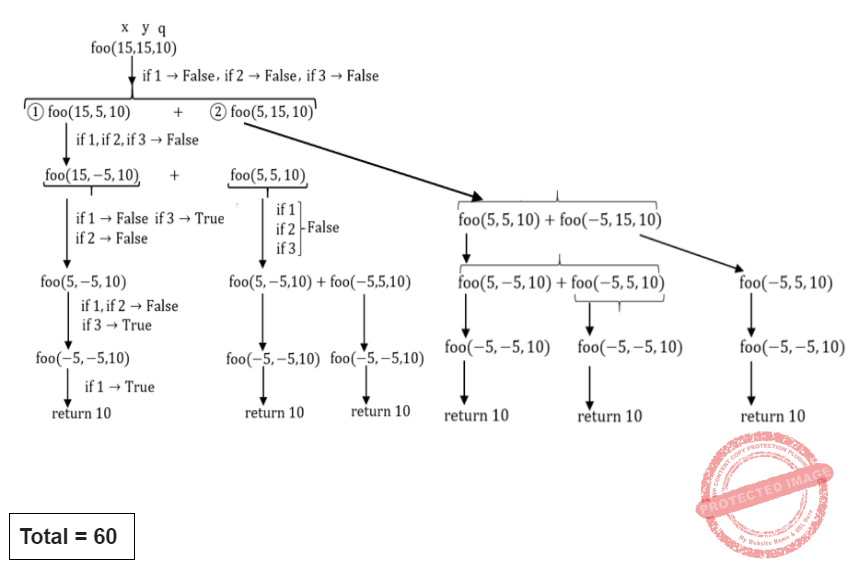There are 16 questions to complete.

Register Now